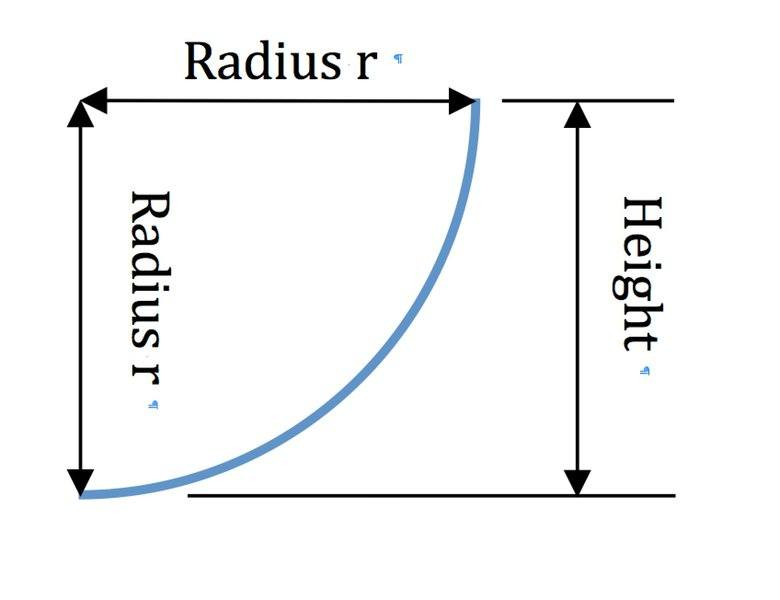# Conservation of energy

## Homework Statement

A block of mass 10kg starts at the top of a frictionless track which forms a quarter circle with radius 10m. It is given an initial downward velocity of 10m/s. What is the velocity at the bottom of the track?

## Homework Equations

KEtranslational, f+ KErotational, f+PEf=KEtranslational, i+KErotational, i+ PEf
KE, rotational=1/2Iw2
KE, translational=1/2mv2
PE=mgh
I=MR2
w=v/r

## The Attempt at a Solution

I believe the initial equation would be set up like this.
1/2mvf2+ 1/2 MR2(V/R)2+mghf=1/2mvi2+1/2 MR2(V/R)2+mghi

which would then reduce to:
vf2=vi2+ghinitial

This does not seem like the likely way to do it because we are not given the height of the mass at the top. Should I be using the rotational and translational KE in the equation? At what point is it rotational and what point translational?

vf2=vi2+ghinitial

This does not seem like the likely way to do it because we are not given the height of the mass at the top.

Look carefully.

Should I be using the rotational and translational KE in the equation? At what point is it rotational and what point translational?

Why would it rotate? When does something rotate?

Look carefully.

Why would it rotate? When does something rotate?

Would the radius be the initial height?

When it is rolling? I'm not exactly sure.

Would the radius be the initial height?

That is correct.

When it is rolling? I'm not exactly sure.

Try and think intuitively if you don't know the physics behind it.

That is correct.

Try and think intuitively if you don't know the physics behind it.
Ok, now I see how to do this. I obtained 17.2 m/s, which is correct. Thank you!

However, I still don't see how the radius could be equal to the height of the ramp. There is a picture provided that looks like a skateboard ramp, with a strict vertical height and then the inside being curved with a radius. The vertical height does not look equal to the radius, even though I know this is not to scale.

Does the picture below look like the picture you have of the skateboard ramp? Is the quarter circle oriented like that?•Mastermind01
Does the picture below look like the picture you have of the skateboard ramp? Is the quarter circle oriented like that?View attachment 197493
yes, it is just like that, except flipped so that the curve is on the right and height on the left.

So does it make sense to you that the radius = height?

Yes, I do see it now-my picture did not have the radius being pointed horizontally and vertically, just down the middle. Thank you for all your help!

•TomHart